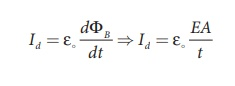Home | | Physics 12th Std | Solved Example Problems on Displacement current and MaxwellŌĆÖs correction to Ampere's circuital law

# Solved Example Problems on Displacement current and MaxwellŌĆÖs correction to Ampere's circuital law

Displacement current and MaxwellŌĆÖs correction to Ampere's circuital law: Solved Example Problems with Answers, Solution and Explanation

## Displacement current and MaxwellŌĆÖs correction to Ampere's circuital law: Solved Example Problems

### EXAMPLE 5.1

Consider a parallel plate capacitor which is maintained at potential of 200 V. If the separation distance between the plates of the capacitor and area of the plates are 1 and 20 cm2. Calculate the displacement current for the time in ┬Ąs.

### Solution

Potential difference between the plates of the capacitor, V = 200 V

The distance between the plates,

d = 1 mm = 1 ├Ś 10-3 m

Area of the plates of the capacitor,

A = 20 cm2 = 20 ├Ś 10-4 m2

Time is given in micro-second, ┬Ąs = 10-6 s

Displacement currentBut electric field, E = V/d

Therefore,= 35400 ├Ś10ŌłÆ7 = 3 .5 mA

Tags : Electromagnetic Waves | Physics , 12th Physics : Electromagnetic Waves
Study Material, Lecturing Notes, Assignment, Reference, Wiki description explanation, brief detail
12th Physics : Electromagnetic Waves : Solved Example Problems on Displacement current and MaxwellŌĆÖs correction to Ampere's circuital law | Electromagnetic Waves | Physics